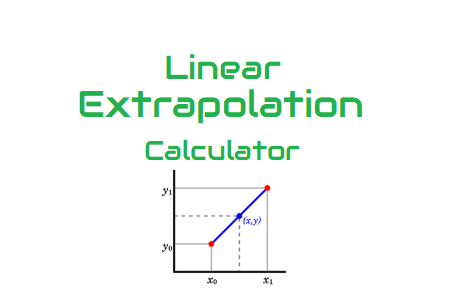# Linear Extrapolation CalculatorIn Performance Testing, when the test environment has a scaled-down ratio like 50%, 25% of production environment then you can not run the test with full load i.e. at production load. In this case, you need to reduce the number of users according to the environment scaled down ratio and execute the test. As per the capacity of the scaled-down environment, create the load test scenario, run the test and then extrapolate the results as per production servers. Refer to the link to understand ‘Extrapolation Method‘. There are 3 methods of extrapolation. Linear, S-Curve and Mixed-mode Extrapolation. Below given Linear Extrapolation Calculator will help you to calculate the linear extrapolation of the performance test metric.

### How to use Linear Extrapolation Calculator?

Linear Extrapolation provides you with a predicted value of a performance metric when a linear behaviour identified in the graph. For example, a gradual increase in throughput identified on increasing the user load on an application. Refer to the below screenshot: